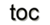Next: 8.1 Visual Programming Up: 4 Applied Concepts Previous: 7.4 Performance Analysis

# 8. Generic Application Design

Generic application design deals with the conceptual categorization of computational domains, the reduction of algorithms to their minimal conceptual requirements, and strict performance guarantees. Here, the assembly of the given practical building blocks (see Part III) and the transformation of GSSE components into actual applications are presented. Several examples are given to demonstrate that the proposed concepts are functional and that the implementation effort is greatly reduced. The application areas are divided into different areas, such as wave equation, diffusion simulation, and electrostatic simulation. A system of coupled non-linear equations from the field of TCAD's device simulation is used to demonstrate the approach, and several results for the investigated equations are presented. All examples use automatic model quantity evaluation, while communication is accomplished using the GFI module. Each of the applications presented is implemented following the same patterns:

• Specification of required quantities by corresponding identifier, e.g., strings.
• Use of a linear or non-linear solution scheme by the selection of the appropriate solver scheme.
• Implementation of pre-processing sections.
• Implementation of the application code.
• Implementation of post-processing sections.

The actual application code requires normally not more than hundreds of source lines for each application, and the pre-processing and post-processing facilities can be reused. Related to the finite element scheme, GSSE contains pre-calculated stencil matrices for triangles, rectangles, tetrahedra, and cubes. Cell topologies (posets) are stored for from one-dimensional up to four-dimensional simplices and cuboids and can thereby be optimized at compile-time. Compile-time as well as run-time algorithms are available to generate higher dimensional posets. Interpolation algorithms are available for linear, quadratic, and exponential methods.

SubsectionsNext: 8.1 Visual Programming Up: 4 Applied Concepts Previous: 7.4 Performance Analysis

R. Heinzl: Concepts for Scientific Computing